# Chamfer (geometry)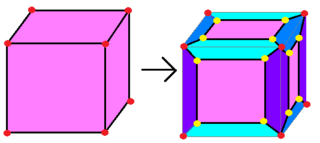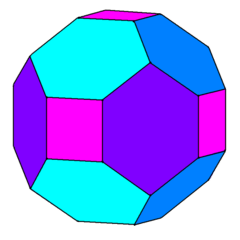A chamfered cube can be constructed by reducing the square faces, and connecting new edges to vertices at the original positions.Topologically: (v,e,f) --> (v+2e,4e,f+e)

In geometry, chamfering or edge-truncation is a Conway polyhedron notation operation that modifies one polyhedron into another. It is similar to expansion, moving faces apart and outward, but also maintain the original vertices. For polyhedra, this operation adds a new hexagonal face in place of each original edge.

A polyhedron with e edges will have a chamfered form containing 2e new vertices, 3e new edges, and e new hexagonal faces.

## Chamfered regular and quasiregular polyhedra

Class Regular Quasiregular
Seed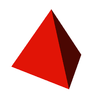{3,3}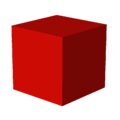{4,3}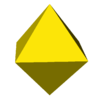{3,4}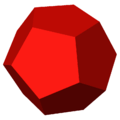{5,3}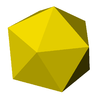{3,5}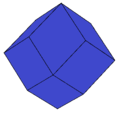aC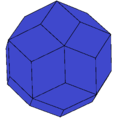Chamfered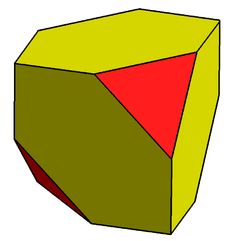cT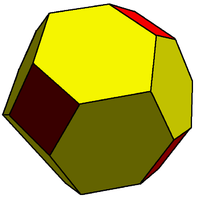cC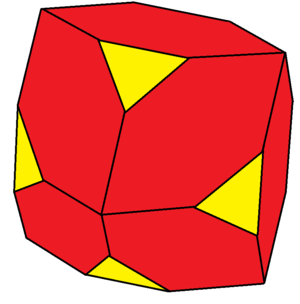cO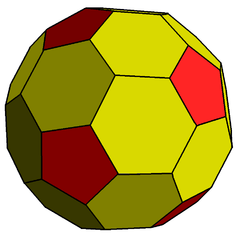cD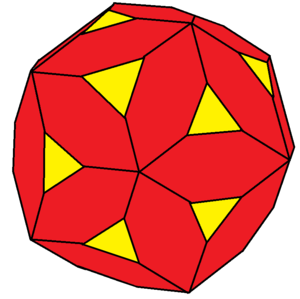cI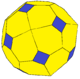caC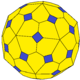## Relation to Goldberg polyhedra

The chamfer operation applied in series creates progressively larger polyhedra with new hexagonal faces replacing edges from the previous one. The chamfer operator transforms G(m,n) to G(2m,2n).

A regular polyhedron, G(1,0), create a Goldberg polyhedra sequence: G(1,0), G(2,0), G(4,0), G(8,0), G(16,0)...

G(1,0) G(2,0) G(4,0) G(8,0) G(16,0)...
GIVCcC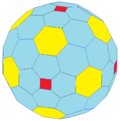ccC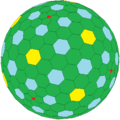cccC
GVDcD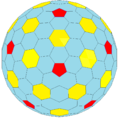ccD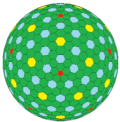cccD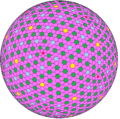ccccD
GVI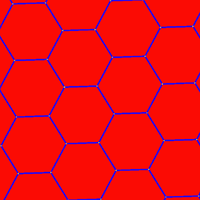H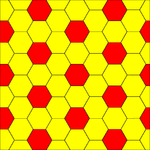cH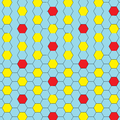ccH

cccH

ccccH

The truncated octahedron or truncated icosahedron, G(1,1) creates a Goldberg sequence: G(1,1), G(2,2), G(4,4), G(8,8)....

G(1,1) G(2,2) G(4,4)...
GIV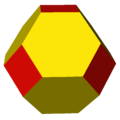tO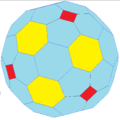ctO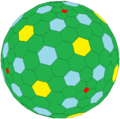cctO
GV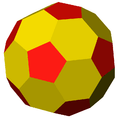tI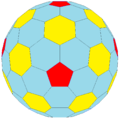ctI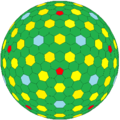cctI
GVI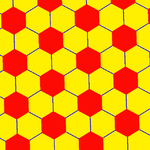tH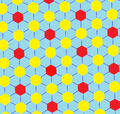ctH

cctH

A truncated tetrakis hexahedron or pentakis dodecahedron, G(3,0), creates a Goldberg sequence: G(3,0), G(6,0), G(12,0)...

G(3,0) G(6,0) G(12,0)...
GIV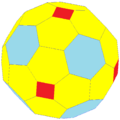tkC
ctkC cctkC
GV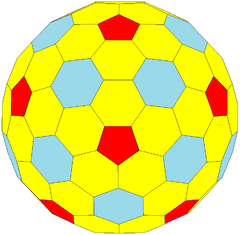tkD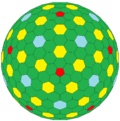ctkD
cctkD
GVI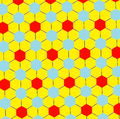tkH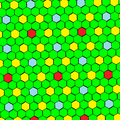ctkH
cctkH

## Chamfered regular tilings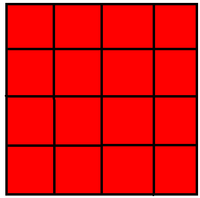Square tiling, Q{4,4}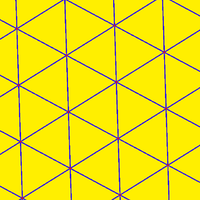Triangular tiling, Δ{3,6}Hexagonal tiling, H{6,3}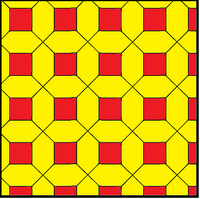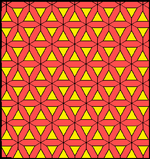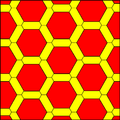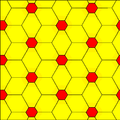cQ cΔ cH

## Chamfered polytopes and honeycombs

Like the expansion operation, chamfer can be applied to any dimension. For polygons, it triples the number of vertices. For polychora, new cells are created around the original edges, The cells are prisms, containing two copies of the original face, with pyramids augmented onto the prism sides.

## Chamfered tetrahedron

Chamfered tetrahedronConway notationcT
Goldberg polyhedronGIII(2,0)
Faces4 triangles
6 hexagons
Edges24 (2 types)
Vertices16 (2 types)
Vertex configuration(12) 3.6.6
(4) 6.6.6
Symmetry groupTetrahedral (Td)
Dual polyhedronAlternate-triakis octahedron
Propertiesconvex, equilateral-faced

The chamfered tetrahedron (or alternate truncated cube) is a convex polyhedron constructed as an alternately truncated cube or chamfer operation on a tetrahedron, replacing its 6 edges with hexagons.

It is the Goldberg polyhedron GIII(2,0), containing triangular and hexagonal faces.

It can look a little like a truncated tetrahedron,, which has 4 hexagonal and 4 triangular faces, which is the related Goldberg polyhedron: GIII(1,1).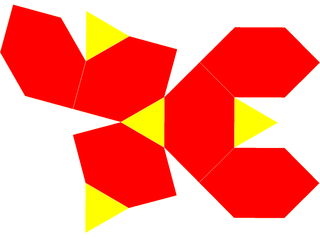Net

## Chamfered cube

Chamfered cubeConway notationcC = t4daC
Goldberg polyhedronGIV(2,0)
Faces6 squares
12 hexagons
Edges48 (2 types)
Vertices32 (2 types)
Vertex configuration(24) 4.6.6
(8) 6.6.6
SymmetryOh, [4,3], (*432)
Dual polyhedronTetrakis cuboctahedron
Propertiesconvex, zonohedron, equilateral-faced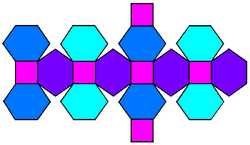net

In geometry, the chamfered cube (also called truncated rhombic dodecahedron) is a convex polyhedron constructed from the rhombic dodecahedron by truncating the 6 (order 4) vertices.

The 6 vertices are truncated such that all edges are equal length. The original 12 rhombic faces become flattened hexagons, and the truncated vertices become squares.

The hexagonal faces are equilateral but not regular. They are formed by a truncated rhombus, have 2 internal angles of about 109.47 degrees () and 4 internal angles of about 125.26 degrees, while a regular hexagon would have all 120 degree angles.

Because all its faces have an even number of sides with 180 degree rotation symmetry, it is a zonohedron. It is also the Goldberg polyhedron GIV(2,0), containing square and hexagonal faces.

### Coordinates

The chamfered cube is the Minkowski sum of a rhombic dodecahedron and a cube of side length 1 when eight vertices of the rhombic dodecahedron are atand its six vertices are at the permutations of.

This polyhedron looks similar to the uniform truncated octahedron:

Chamfered cubeTruncated octahedron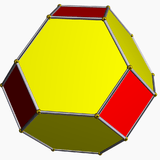We can construct a truncated octahedron model by twenty four chamfered cube blocks.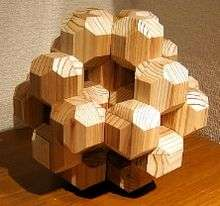## Chamfered octahedron

Chamfered octahedronConway notationcO = t3daO
Faces8 triangles
12 hexagons
Edges48 (2 types)
Vertices30 (2 types)
Vertex configuration(24) 3.6.6
(6) 6.6.6
SymmetryOh, [4,3], (*432)
Dual polyhedronTriakis cuboctahedron
Propertiesconvex

In geometry, the chamfered octahedron is a convex polyhedron constructed from the rhombic dodecahedron by truncating the 8 (order 3) vertices.

The 8 vertices are truncated such that all edges are equal length. The original 12 rhombic faces become flattened hexagons, and the truncated vertices become triangles.

The hexagonal faces are equilateral but not regular.

## Chamfered dodecahedron

Chamfered dodecahedronGoldberg polyhedronGV(2,0)
Fullerene C80
Faces12 pentagons
30 hexagons
Edges120 (2 types)
Vertices80 (2 types)
Vertex configuration(60) 5.6.6
(20) 6.6.6
Symmetry groupIcosahedral (Ih)
Dual polyhedronPentakis icosidodecahedron
Propertiesconvex, equilateral-faced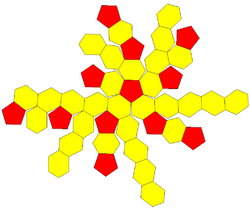The chamfered dodecahedron (also called truncated rhombic triacontahedron) is a convex polyhedron constructed as a truncation of the rhombic triacontahedron. It can more accurately be called a pentatruncated rhombic triacontahedron because only the order-5 vertices are truncated.

These 12 order-5 vertices can be truncated such that all edges are equal length. The original 30 rhombic faces become non-regular hexagons, and the truncated vertices become regular pentagons.

The hexagon faces can be equilateral but not regular with D2 symmetry. The angles at the two vertices with vertex configuration 6.6.6 are arccos(-1/sqrt(5)) = 116.565 degrees, and at the remaining four vertices with 5.6.6, they are 121.717 degrees each.

It is the Goldberg polyhedron GV(2,0), containing pentagonal and hexagonal faces.

This polyhedron looks very similar to the uniform truncated icosahedron which has 12 pentagons, but only 20 hexagons.

It also represents the exterior envelope of a cell-centered orthogonal projection of the 120-cell, one of six (convex regular 4-polytopes).

### Chemistry

This is the shape of the fullerene C80; sometimes this shape is denoted C80(Ih) to describe its icosahedral symmetry and distinguish it from other less-symmetric 80-vertex fullerenes. It is one of only four fullerenes found by Deza, Deza & Grishukhin (1998) to have a skeleton that can be isometrically embeddable into an L1 space.

## Chamfered icosahedron

Chamfered icosahedronConway notationcI = t3daI
Faces20 triangles
30 hexagons
Edges120 (2 types)
Vertices72 (2 types)
Vertex configuration(24) 3.6.6
(12) 6.6.6
SymmetryIh, [5,3], (*532)
Dual polyhedrontriakis icosidodecahedron
Propertiesconvex

In geometry, the chamfered icosahedron is a convex polyhedron constructed from the rhombic triacontahedron by truncating the 20 order-3 vertices. The hexagonal faces can be made equilateral but not regular.